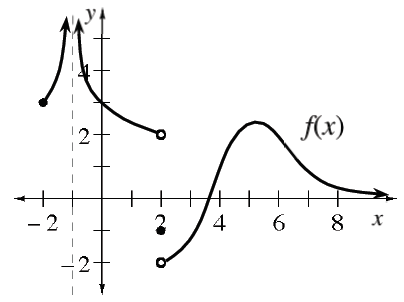### Home > APCALC > Chapter 4 > Lesson 4.2.3 > Problem4-72

4-72.

The graph of a function $y = f\left(x\right)$ is shown at the right. Use the graph to evaluate the following limits.

1. $\lim\limits _ { x \rightarrow - 1 } f ( x )$

The limit does not exist, but $y→+∞$.

2. $\lim\limits _ { x \rightarrow 2 } f ( x )$

A limit is a predicted value (which sometimes differs from the actual function value). The prediction must agree from the left and the right.

3. $\lim\limits _ { x \rightarrow 2 ^ { - } } f ( x )$

What is the prediction from the left?

4. $\lim\limits _ { x \rightarrow 2 ^ { + } } f ( x )$

What is the prediction from the right?

5. $\lim\limits _ { x \rightarrow 5 } f ( x )$

Most of the time, the limit and the function value agree.

6. $\lim\limits _ { x \rightarrow \infty } f ( x )$

$\lim\ x→∞$ and $\lim\ x→−∞$ will reveal the equation of a horizontal asymptote, if there is one.

7. Where (if anywhere) does the derivative of $f$ not exist?

Look for cusps, endpoints, jumps, holes, and vertical asymptotes.